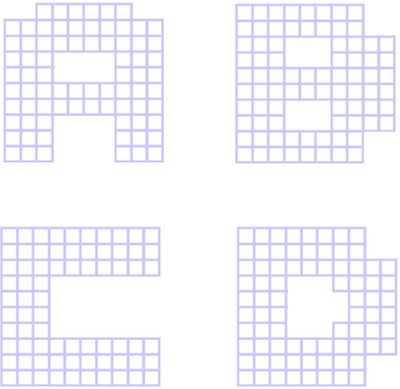# The Square Counting Shortcut

Alignments to Content Standards: 3.MD.C

Imagine that each square in the picture measures one centimeter on each side. What is the area of each letter? Try to work it out without counting each square individually.## IM Commentary

This is a rectangle subdivision task; ideally instead of counting each square. students should break the letters into rectangles, multiply to find the areas, and add up the areas. However, students should not be discouraged from using individual counting to start if they are stuck. Often students will get tired of counting and devise the shortcut method themselves.

It is acceptable to mix rectangle-area counting and traditional counting, like D in the example solution demonstrates.

Students may also intuitively exploit symmetry to help with the problem, although symmetry is not introduced in the standards until Grade 4.

The Standards for Mathematical Practice focus on the nature of the learning experiences by attending to the thinking processes and habits of mind that students need to develop in order to attain a deep and flexible understanding of mathematics. Certain tasks lend themselves to the demonstration of specific practices by students. The practices that are observable during exploration of a task depend on how instruction unfolds in the classroom. While it is possible that tasks may be connected to several practices, only one practice connection will be discussed in depth. Possible secondary practice connections may be discussed but not in the same degree of detail.

This particular task helps illustrate Mathematical Practice Standard 8, Look for and express regularity in repeated reasoning. Third graders might begin to individually count the square units but will quickly notice that there are similar shapes in each of these letter configurations. They will build upon this observation of visible rectangles to find shortcut ways of determining the area of each configuration without counting every rectangle. Most students will use a combination of area calculations for larger rectangular blocks of squares, and simply counting squares for other parts as is shown in the solution method for “D.” Some students may choose to use an additive perspective or other students may visualize the whole as a square and remove rectangles to determine total area. When students are engaged in MP.8, they purposely look for patterns/similarities, make conjectures about these patterns/similarities, consider generalities and limitations, and make connections over time about their ideas.  They pay special attention to repeated logic that might be simplified to arrive at the solution more efficiently.  As they begin to explain their processes to one another, they construct, critique, and compare arguments (MP.3).

Submitted by Jason Dyer to the fourth Illustrative Mathematics task writing contest.

## Solutions

Solution: Solution

(There are many ways to subdivide the letters into rectangles, this is one solution of many.)The letter "A" has an area of 76 square cm.

The letter "B" has an area of 80 square cm.

The letter "C" has an area of 72 square cm.

The letter "D" has an area of 78 square cm.

Solution: Using subtraction

Another way students might do this is to figure out the number of squares that have been "removed" from a square that is 10 cm on each side.A square that is 10 cm on each side has an area of 100 square cm.

\begin{align} 100 - (1\times 2 + 1\times2 + 2 \times 4 + 3\times 4) &= 100 - (2 + 2 + 8 + 12) \\ &= 100 - 24 \\ &= 76 \end{align}

So the area of the letter A is 76 square centimeters. These others can be done in a similar manner.